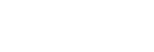# Formula and Equations For Capacitor and Capacitance

## Capacitor and Capacitance Formulas and Equations

The following formulas and equations can be used to calculate the capacitance and related quantities of different shapes of capacitors as follow.### Capacitance of Capacitor:

The capacitance is the amount of charge stored in a capacitor per volt of potential between its plates. Capacitance can be calculated when charge Q & voltage V of the capacitor are known:

C = Q/V

#### Charge Stored in a Capacitor:

If capacitance C and voltage V is known then the charge Q can be calculated by:

Q = C V

#### Voltage of the Capacitor:

And you can calculate the voltage of the capacitor if the other two quantities (Q & C) are known:

V = Q/C

Where

• Q is the charge stored between the plates in Coulombs
• C is the capacitance in farads
• V is the potential difference between the plates in Volts

#### Reactance of the Capacitor:

Reactance is the opposition of capacitor to Alternating current AC which depends on its frequency and is measured in Ohm like resistance. Capacitive reactance is calculated using:Where

• Xis the capacitive reactance
• F is the applied frequency
• C is the capacitance

#### Quality Factor of Capacitor:

Q factor or Quality factor is the efficiency of the capacitor in terms of energy losses & it is given by:

QF = XC/ESR

Where

• Xis the capacitive reactance
• ESR is the equivalent series resistance of the capacitor.

#### Dissipation Factor of Capacitor:

D factor or dissipation factor is the inverse of the Quality factor, it shows the power dissipation inside the capacitor & is given by:

DF = tan δ = ESR/XC

Where

• DF is the dissipation factor
• δ is the angle between capacitive reactance victor & negative axis.
• Xis the capacitive reactance
• ESR is the equivalent series resistance of the circuit.

Related Posts:

### Energy Stored in a Capacitor:

The Energy E stored in a capacitor is given by:

E = ½ CV2

Where

• E is the energy in joules
• C is the capacitance in farads
• V is the voltage in volts

#### Average Power of Capacitor

The Average power of the capacitor is given by:

Pav = CV2 / 2t

where

• t is the time in seconds.

### Capacitor Voltage During Charge / Discharge:

When a capacitor is being charged through a resistor R, it takes upto 5 time constant or 5T to reach upto its full charge. The voltage at any specific time can by found using these charging and discharging formulas below:

#### During Charging:

The voltage of capacitor at any time during charging is given by:#### During Discharging:

The voltage of capacitor at any time during discharging is given by:Where

• VC is the voltage across the capacitor
• Vs is the voltage supplied
• t  is the time passed after supplying voltage.
• RC = τ is the time constant of the RC charging circuit

Related Posts:

### Capacitance Formulas

The capacitance between two conducting plates with a dielectric between then can be calculated by:• k is the dielectric constant
• εis the permittivity of the dielectric
• ε0 ­­is the permittivity of space which is equal to 8.854 x 10-12 F/m
• A is the area of the plates
• d is the separation between the plates

#### Capacitance of a Plate Capacitor FormulaWhere:

• C is capacitance in farads
• A is the plat area
• n is the number of plates
• d is the plate separation distance
• εr is the relative permeability of the substance between the plates
• εo absolute permittivity

#### Self Capacitance of a Coil (Medhurst Formula)

C2 ≈ (0.256479 h2 + 1.57292 r2) pF

Where:

• h2 and r2 in inches

#### Self Capacitance of a Sphere Formula

C2b ≈ 4πεor

Where:

• r is the radius of sphere

#### Self Capacitance of a Toroid Inductor Formula

• r is the small radius
• R is the large radius

#### Ohm’s Law for Capacitor:

Q = CV

By differentiating the equation, we get:where

• i is the instantaneous current through the capacitor
• C is the capacitance of the capacitor
• Dv/dt is the instantaneous rate of change of voltage applied.

Related Formulas and Equations Posts: